# Vectors

A vector is a direction and magnitude (length). Vectors are used to represent many different things - light source directions, surface orientations, relative distances between objects, etc.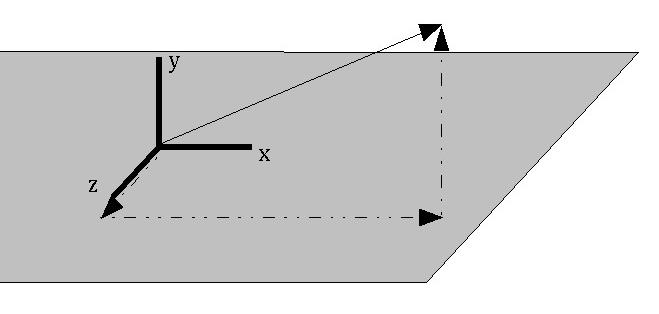# Vectors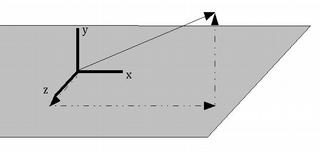We normally describe a vector as a triplet of (X, Y, Z) values - (vx, vy, vz) represents a vector that points vx units in the direction of the X axis, vy units in the direction of the Y axis, and vz units in the direction of the Z axis.

e.g., (2, 0, 0) is a vector pointing in the direction of the X axis, 2 units long. (1, 1, 0) is a vector pointing at a 45 degree angle between the X and Y axes, 1.414 units long.

The magnitude of a vector (x,y,z) is its Euclidean length - the square root of vx2 + vy2 + vz2.

Two vectors can be combined by adding their corresponding components together.
i.e. (vx, vy, vz) + (wx, wy, wz) is (vx+wx, vy+wy, vz+wz ).

Or, written more expansively:

```  | vx |     | wx |     | vx + wx |
| vy |  +  | wy |  =  | vy + wy |
| vz |     | wz |     | vz + wz |
```

The result is a vector that is equivalent to sticking the vector W onto the end of vector V, and creating a new vector from the beginning of V to the end of W.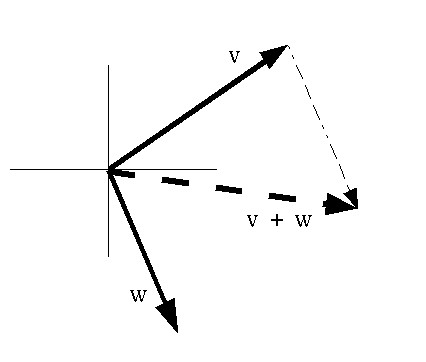# Vector/Scalar Multiplication

A vector can be multiplied by a single number (a "scalar") to change its length without changing its direction.

```        | vx |     | s * vx |
s  *  | vy |  =  | s * vy |
| vz |     | s * vz |
```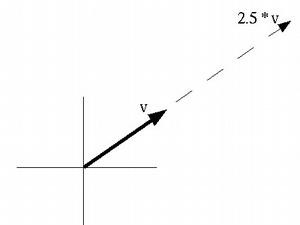# Dot Product

The dot product of two vectors is an operation defined as:

```  | x0 |   | x1 |
| y0 | * | y1 |  =  x0*x1 + y0*y1 + z0*z1
| z0 |   | z1 |
```
The result is a single number, which is equal to the product of the lengths of the two vectors and the cosine of the angle between them.

It can tell us how much two vectors point in the same direction - it is maximum when they point in exactly the same direction, and it's 0 when they're at right angles.

# Cross Product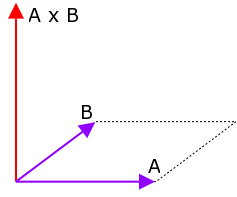The cross product is a function of two vectors that will produce a new vector perpendicular to both. The length of the cross product is equal to the area of the parallelogram formed by the two vectors.

The formula for the cross product of two vectors A & B is:

[ ( Ay Bz - Az By ),   ( Az Bx - Ax Bz ),   ( Ax By - Ay Bx ) ]

# Cross Product

The Python equivalent is:

```def crossProduct(A,B):
return [ A * B - A * B,
A * B - A * B,
A * B - A * B ]
```

The direction of the cross product is determined by the right-hand rule applied from vector A to vector B (so if you multiply them in the reverse order, the result will point the opposite direction).

# Motion With Vectors

A vector can be used to represent the motion of an object.

The vector indicates the object's velocity - the direction of the vector is the direction that the object is moving, the magnitude of the vector is the speed.

The vector corresponds to how the object will move in one unit of time.
e.g., a velocity vector of (2, 0, 0) indicates that the object is moving 2 meters/second along the X axis (assuming our units are meters and seconds). If it starts at (3, 1, 0), then after 1 second its position will be (5, 1, 0). After 2 seconds, its position will be (7, 1, 0).

[Note that the choice of units is arbitrary, and is up to you when writing your programs; all that matters is that you be consistent within a program, and not, for instance, try to combine meters per second with miles per hour.]

# Motion With Vectors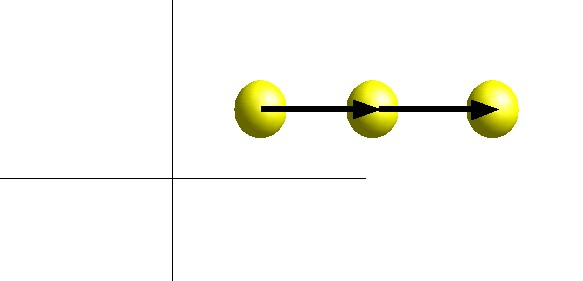In general, if an object starts at position P0, with velocity V, then after t time units, its position P(t) will be:

```            P(t) = P0 + t * V
```

# Programming With Vectors

Creating a vector class allows one to work with vectors more simply.

Vector operations just require a single statement, rather than a loop or separate statements for X, Y, & Z components.

```    a = Vector((1, 2, 3))
b = Vector((0, -1, 0))
c = a + 2 * b
d = a.dot(b)
```

# dmsgl Utilities

dmsgl.py

Misc functions:

• drawStrokeString
• drawBitmapString
• drawAxes
Classes:
• Vector
• Color - derived from Vector
• Texture2D
• Camera
• PerspCamera & OrthoCamera - derived from Camera
• WFObject

Python's open() function opens a file for reading (or writing)

file.readlines() reads the entire contents of file into a list of strings

```    inputfile = open("data.txt", "r")
inputfile.close()

for line in data:
print line
```

The string package contains functions for manipulating strings

string.split() breaks up a string, based on a separating character, into a list of strings

string.atoi() converts a string to an integer
string.atof() converts a string to a floating point number

```    import string

for line in data:
strvalues = string.split(line)
for str in strvalues:
x = string.atoi(str)
print x
```

# OBJ Format

An OBJ file is a plain text file, containing vertices, polygon faces, and other information. Each vertex, face, etc, is given on a separate line.

Each line begins with a token to identify what sort of line it is - 'v' for vertex, 'f' for face, etc.

v x y z
vertex position
vn x y z
vertex normal
vt u v
texture coordinate
f v1 v2 v3 ...
face (list of vertex numbers)
mtllib file.mtl
file containing material descriptions
usemtl name
current material to apply to geometryThis document is by Dave Pape, and is released under a Creative Commons License.